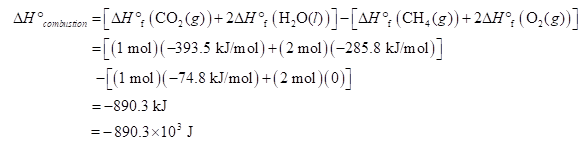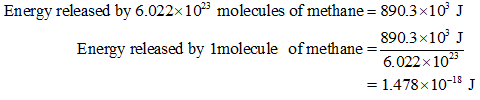# Consider the combustion of a single molecule of CH4(g), forming H2O(l) as a product.How much energy in J, is produced during this reaction?A typical X-ray light source has an energy of 8 keV. Is the energy released by the combustion of a CH4 molecule larger or smaller than the energy of an X-ray from this source?

Question
4 views
• Consider the combustion of a single molecule of CH4(g), forming H2O(l) as a product.
1. How much energy in J, is produced during this reaction?
2. A typical X-ray light source has an energy of 8 keV. Is the energy released by the combustion of a CH4 molecule larger or smaller than the energy of an X-ray from this source?
check_circle

Step 1

Heat released in a reaction can be calculated from Hess's law by subtracting the  enthalpies of formation of reactants from that of products.

Step 2

1)

Complete combustion of methane takes place by the equation:

CH4 (g) + O2 (g) -----> CO2 (g) + H2O (l)

The standard enthalpies of formation of CH4 (g) , O2 (g), CO2 (g) and H2O (l) are -74.8 kJ/mol, 0 kJ/mol, -393.5 kJ/mol and -285.8 kJ/mol respectively.

Calculate the enthalpy of combustion of methane as shown below:Step 3

890.3 x 103 J of energy is released by combustion of one mol (6.022 x 1023 molecules) of methane.

Energy released by combustion of 1 molecule can be calculated as:...

### Want to see the full answer?

See Solution

#### Want to see this answer and more?

Solutions are written by subject experts who are available 24/7. Questions are typically answered within 1 hour.*

See Solution
*Response times may vary by subject and question.
Tagged in

### Chemistry